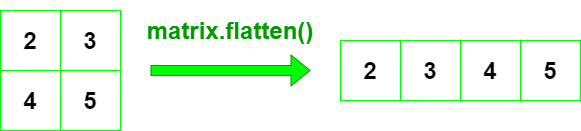Open in App
Not now

# Flatten a Matrix in Python using NumPy

• Last Updated : 29 Aug, 2020

Let’s discuss how to flatten a Matrix using NumPy in Python. By using ndarray.flatten() function we can flatten a matrix to one dimension in python.

Syntax:numpy_array.flatten(order=’C’)

• order:‘C’ means to flatten in row-major.’F’ means to flatten in column-major.’A’ means to flatten in column-major order if a is Fortran contiguous in memory, row-major order otherwise.’K’ means to flatten a in the order the elements occur in memory. The default is ‘C’.

Return:Flattened 1-D matrixExample 1:

## python3

 `# importing numpy as np``import` `numpy as np`` ` `# declare matrix with np``gfg ``=` `np.array([[``2``, ``3``], [``4``, ``5``]])`` ` `# using array.flatten() method``flat_gfg ``=` `gfg.flatten()``print``(flat_gfg)`

Output:

```[2 3 4 5]
```

Example 2:

## python3

 `# importing numpy as np``import` `numpy as np`` ` `# declare matrix with np``gfg ``=` `np.array([[``6``, ``9``], [``8``, ``5``], [``18``, ``21``]])`` ` `# using array.flatten() method``gfg.flatten()`

Output:

```array([ 6,  9,  8,  5, 18, 21])
```

Example 3:

## python3

 `# importing numpy as np``import` `numpy as np`` ` `# declare matrix with np``gfg ``=` `np.array([[``6``, ``9``, ``12``], [``8``, ``5``, ``2``], [``18``, ``21``, ``24``]])`` ` `# using array.flatten() method``flat_gfg ``=` `gfg.flatten(order``=``'A'``)``print``(flat_gfg)`

Output:

```[ 6,  9, 12,  8,  5,  2, 18, 21, 24]
```

My Personal Notes arrow_drop_up